Previous page

16. The Thermistor

The resistance of a thermistor falls when the temperature rises.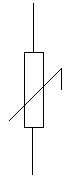17. Binary numbers

A binary number is a number in base 2. You remember in primary school, where above each sum you put H, T, & U for Hundreds, Tens, & Units? Well, binary is similar to that. But binary only uses 1 & 0.

 32 16 8 4 2 1 1 0 1 0 1 1 32 + 8 + 2+ 1

Using the above, we can see that 101011 = 43 (32+8+2+1).

We transform a decimal (Base 10 - normal numbers) to binary in a similar fashion to a long division. We write the headers (1, 2, 4, etc. - they double each time, & don't forget to write them down with the smallest on the right) down first, and then we see how many of N will fit into one of our headers.

23 =

 32 16 8 4 2 1 0 1 0 1 1 1

Note that it's possible to count on binary on your fingers - just use an extended finger to represent 1, & a closed finger to represent 2. On one hand, you can count up to 31, & with both hands, you can count up to 1023!

Binary counting

 0 0000 1 0001 2 0010 3 0011 4 0100 5 0101 6 0110 7 0111 8 1000 9 1001 10 1010 11 1011 12 1100 13 1101 14 1110 15 1111

Note that the zeros before the first 1 are not required, but I have put them in to demonstrate the pattern. To add binary numbers, we needed an arrangement as follows: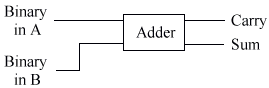We need to design a logic circuit to do this for us, from the truth table below (S = sum. C = carry). Note that this is a very good test to check that you're good at logic, so why not try & design the circuit from the truth table without looking at the logic circuit?

 A B S C 0 0 0 0 0 1 1 0 1 0 1 0 1 1 0 1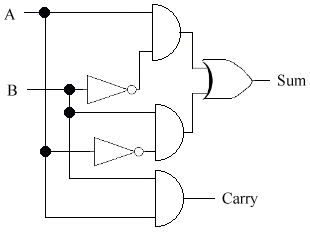This circuit is called a half-adder, because it has 2 outputs - a carry, and a sum, & it cannot do anything with the carry part.

18. The seven-segment display

The seven segment display is the standard LCD or LED display for numerals. It is what you have on your watch, microwave, alarm clock, etc.

Why it is called a seven-segment display I don't know, as all the ones I have seen actually have 8 segments - the decimal point. In the diagram below, I have marked the decimal point for the readers in which their chief interest is to learn electronics, rather than pass an exam. For the exam, however, you do not need to know about the decimal point.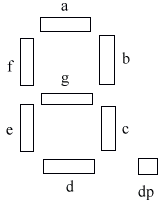All decimal numbers can be achieved by illuminating the right segments.

The seven segment display is wired as follows: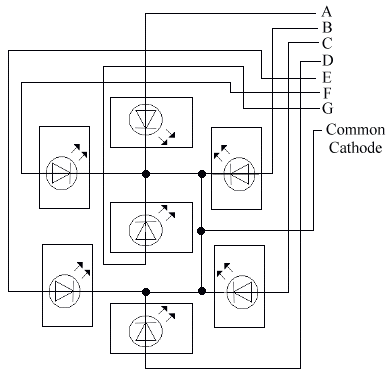Trust me. It's not as complicated as it looks. Try following the letters back to the segments.

19. The Binary Coded Decimal (BCD)

The binary coded decimal counts in tens.This makes it a lot easier to convert to a seven-segment display.

 0 0000 1 0001 2 0010 3 0011 4 0100 5 0101 6 0110 7 0111 8 1000 9 1001 10 0000 (+1 to carry)

For example, 10 = 0001 0000, 15 = 0001 0101, 20 = 0010 0000, 285 = 0010 1000 0101

To display this on a seven-segment display, we need to use a systems diagram like this one: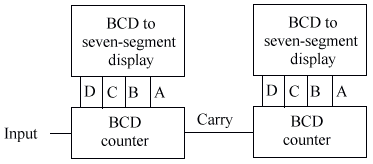20. Diodes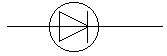A diode only allows current to flow one way. Below is the current-voltage characteristics of different diodes. Here are some of the terms you may see:

Peak Inverse Voltage (PIV) = Maximum reverse voltage allowed.

Forward current rating = maximum forward current allowed.

Silicon diode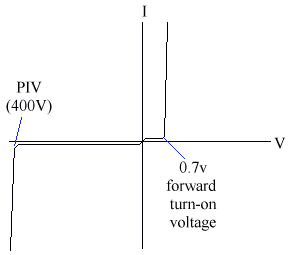There is a forward turn on voltage - The silicon diode will only allow current through it if there is 0.7V flowing through it (i.e. it requires 0.7V to turn itself on). Note that it takes 0.7V out of the supply, so if you have a supply voltage of 6V, & a diode correctly inserted in the circuit, the voltage after the diode will only be 5.3V, because it has taken 0.7V.

Note also that the diode has a peak inverse voltage - There is normally no current flowing through a diode in reverse.

Germanium diode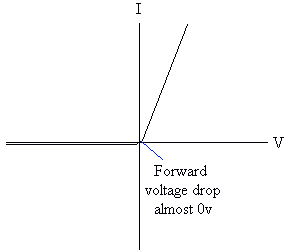Zener diode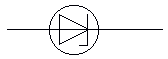These are specially made with low peak inverse voltages. They are used to give a reference voltage, also sometimes called a zener voltage.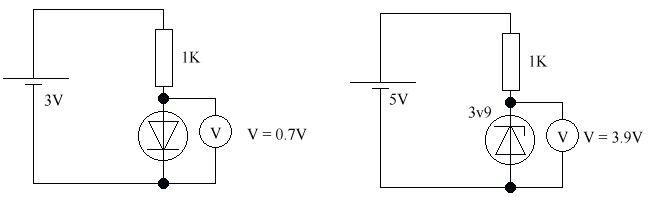The p.d. over the diode is always 3.9v, provided the battery voltage is more than 3.9v. This gives a stable 3.9 v.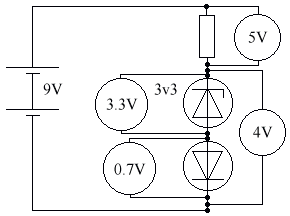The Light Emitting Diode (LED)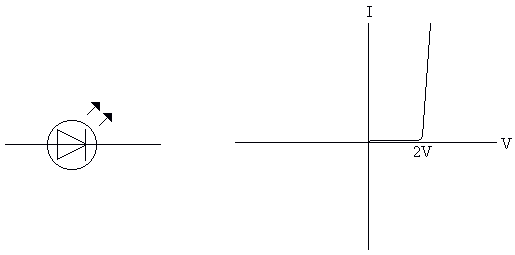The Light Emitting Diode (LED) is made of a different material. Its forward voltage drop is 2v, & the maximum current is about 50 mA. It requires a series resistance to limit the current. The LED is generally used as an indicator, as it doesn't give off enough light to be used as a light source.

For example, to run an LED from a 6V battery at 5mA: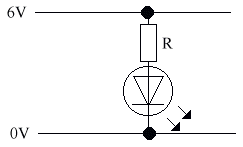R = V / I = (6-2) / 5 = 0K8 = 800R

Use of diodes

• To turn an alternating current (AC) to a direct current (DC).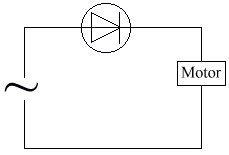• To protect against incorrect polarity.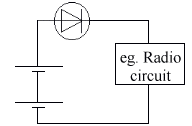• To limit the voltage.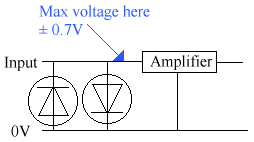Testing diodes

To test a diode, we use an ohmmeter.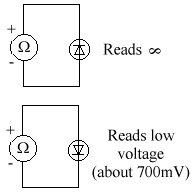Next page